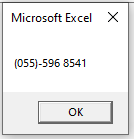# VBA Double Data Type (Dim Variable)

## Double Variable Type

The VBA Double data type is used to store numbers that require decimal places.  It can store from -1.79769313486231E308 to -4.94065645841247E-324 for negative values, and 4.94065645841247E-324 to 1.79769313486232E308 for positive values.

To declare an Double variable, you use the Dim Statement (short for Dimension):

``````Dim dblA as Double
``````

Then, to assign a value to a variable, simply use the equal sign:

``dlbA = 3658.25``

Putting this in a procedure looks like this:

``````Sub dblExample()
'declare the double variable
Dim dblA as Double
'populate the double variable
dblA = 3658.25
'show the message box
MsgBox dblA
End Sub``````

If you run the code above, the following message box will be shown.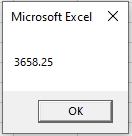## Single Data Type

The Single data type is just a shorter version of the Double data type.  Due to this fact, it can effect the rounding when used in a procedure as the single data type will round to 4 decimal places, while the Double data Type will round to 12 decimal places.  If you do not need the Double data type, you can use the Single data type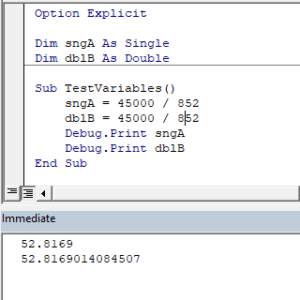## Int or Long Data Types

If you do not need a decimal place, you can use either the Int data type or the Long data type.

``Dim intA as Integer``
``Dim lngB as Long``

## Declare Double Variable at Module or Global Level

In the previous examples, we’ve declared the Double variable within a procedure. Variables declared with a procedure can only be used within that procedure.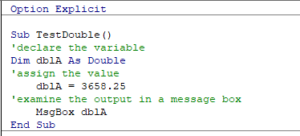Instead, you can declare Double variables at the module or global level.

### Module Level

Module level variables are declared at the top of code modules with the Dim statement.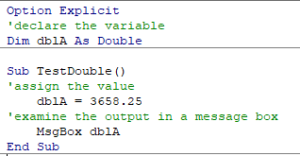These variables can be used with any procedure in that code module.

### Global Level

Global level variables are also declare at the top of code modules. However, instead of using the Dim statement, use the Public statement to indicate that the Double variable is available to be used throughout your VBA Project.

``Public DblA as Double``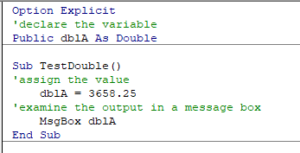If you were to declare the double variable at a module level and then try to use it in a different module, an error would occur.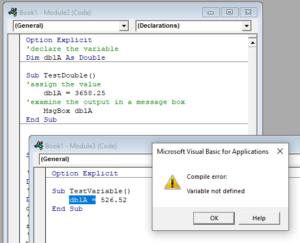However, if you had used the Public keyword to declare the double variable, the error would not occur and the procedure would run perfectly.

Stop searching for VBA code online. Learn more about AutoMacro - A VBA Code Builder that allows beginners to code procedures from scratch with minimal coding knowledge and with many time-saving features for all users!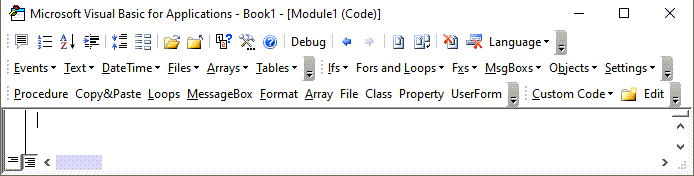## Format Double Stored as String

There may be a time where you wish to format a double data type to a string – for example you might want to display a currency symbol and round the number to 2 decimal places.

To achieve this, you use the Format function.

The  following procedure

``````Sub TestDoubleToCurrencyString()
'declare the string variable
Dim strMoney As String
'declare the double and populate the value
Dim dblValue As Double
dblValue  = 44055.256
'convert the double to a string with a currency symbol with 2 decimal places
strMoney = Format(dblValue , "\$#,##0.00")
'view the result
MsgBox strMoney
End Sub``````

would return this result: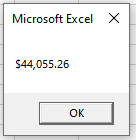Similarly, you may want to display a number as a formatted phone number.

This procedure:

``````Sub TestDoubleToPhone()
'declare the string variable
Dim strPhone As String
'declare the double and populate the value
Dim dblValue As Double
dblValue = 555968541
'convert the double to a string with a currency symbol
strPhone = Format(dblValue, "(000)-000 0000")
'view the result
MsgBox strPhone
End Sub``````

would return this result: